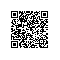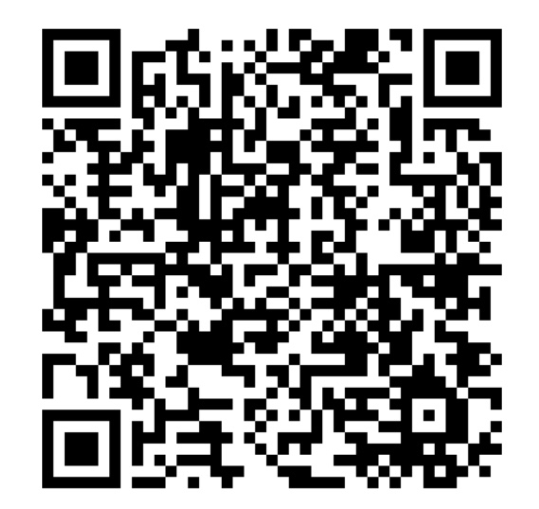# 语言相关-数据类型

10 * 20 等价于 数字 200 (* 是乘法运算符)

10 * "20" 等价于 数字 200

"10" * "20" 等价于 数字 200

10 & 20 等价于 字符串 "1020" (& 是字符串连接运算符)

10 * "fgh" 等价于数值 0.

NOT "" 结果为 布尔值 真 (true).

## 数字类型

(说明:字母e或E后面的数表示指数,因此上例等价于1.5*10*10*10,字母e或E之前必须要有数字,而且需用小数表示,e或E后面的指数必须是整数)

## 字符串类型(String)

"这里是一对 ""双引号"" - ok?"

'这个 "句子" 中包含了 "很多" 的 "双引号" ,留意到了吗?'

"这个 ""句子"" 中包含了 ""很多"" 的 ""双引号"". 能留意到吗?"

## 布尔值(Booleans)

$Boolean1 = true$Boolean2 = false
$Boolean3 =$Boolean1 AND $Boolean2 结果:$Boolean3 为 false

$Boolean1 = false$Boolean2 = not $boolean1 结果:$Boolean2 为 true

$Number1 = 0$Boolean1 = true
$Boolean2 =$Number1 and $Boolean1 (如果含有失败)这个结果将会返回false 如果您计算一个布尔值的算术.(这是不明智的!), 将会返回下面的结果: 如果布尔值为 true 将会转换为数值 1 如果布尔值为 false 将会转换为数值 0 例子:$Boolean1 = true
$Number1 = 100$Number2 = $Boolean1 +$Number1

$String1="Test is: "$String2=$String1 &$Boolean1

## 二进制值(Binary)

Binary 类型可以储存任意字节的值. 当储存时会自动转换为十六进制值(HEX). 例子:
$bin = Binary("abc")$str = String(\$bin)    ; "0x616263"

## 数量类型范围

 数据子类型 范围与说明 Int32 32位浮点整数. Int64 64位浮点整数 Double 双精度浮点数. String 可以包含 2147483647 个字符的字符串. Binary 二进制数据, 可以包含 2147483647 字节数据. Pointer 内存地址指针.  32bit 或者 64bit 定义于 使用的 AutoIt 版本.使用钉钉扫一扫加入圈子
+ 订阅